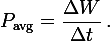# A roller coaster car question

Hello

I have a problem with this exam question does anyone now the solution.

## Homework Statement

A roller coaster car has a mass of 800 kg.
The gravitational field strength is 10 N/kg.
It takes 20 s for the car to reach K from the start.
What is the average power required to raise the car to K in this time?
[Ignore frictional forces]

Hello

I have a problem with this exam question does anyone now the solution.

## Homework Statement

A roller coaster car has a mass of 800 kg.
The gravitational field strength is 10 N/kg.
It takes 20 s for the car to reach K from the start.
What is the average power required to raise the car to K in this time?
[Ignore frictional forces]

Do you have any other data because i believe you would need an angle.

Last edited:
Remember that:BruceW
Homework Helper
Think about the energy of the system. What are the possible forms of energy in this situation (and what equations do you know for them?)

Simply just use:

$$P_{avg}=\frac{\Delta W_{w}}{\Delta t}$$

and in your case the only work applied on the roller coaster is its weight so you will have:

$$P_{avg}=\frac{W_{w}}{\Delta t}$$

Ps: $$W_{w}=mgh$$

Find the gravitational potention energy first
peg=mgh=800x10x20=160000
peg equals to kinect energy at the start because it stops at k
so kinect energy =1/2mvi^2
1/2(800)vi^2=160000
400vi^2=160000
vi= square root of 400
vi=20m/s

power=mv=(800)(20)=16000w

BruceW
Homework Helper
Too much help from you guys. Especially jfxue! The idea of the homework section is to tell the person if they got something wrong or correct and to guide the person by asking them the kinds of questions which will get them thinking in the right direction.

Find the gravitational potention energy first
peg=mgh=800x10x20=160000
peg equals to kinect energy at the start because it stops at k
so kinect energy =1/2mvi^2
1/2(800)vi^2=160000
400vi^2=160000
vi= square root of 400
vi=20m/s

power=mv=(800)(20)=16000w

But you can't say it equals the kinetic energy at the start even though Em(i)=Em(k).

He doesn't have to go looking for all of that stuff. All he has to do is find the work from the start to K then use it to find the average power.

thats not alot of stuff what you mean finding the work from the start how can u find the work from the start without knowing the vi or distance. my way isnt even long

BruceW
Homework Helper
jfxue, I think you've read the question wrong. The car gains GPE due to an electric motor. The question is asking for the work done, so in fact, we must assume the initial KE is zero. (Or account for the KE in our calculation of the power, but that just makes it unnecessarily complicated).

Edit: I didn't explain very well. I'm just trying to say that the question is about the work required to get the car up the hill. This work is done by a motor, but that doesn't even matter really, since we are simply trying to find the average power over the time specified, as mtayab has said.

Last edited: